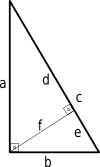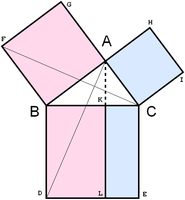# Right triangleRight triangle

A right triangle (American English) or right-angled triangle (British English) is a triangle in which one angle is a right angle (that is, a 90-degree angle). The relation between the sides and angles of a right triangle is the basis for trigonometry.

The side opposite the right angle is called the hypotenuse (side c in the figure). The sides adjacent to the right angle are called legs (or catheti, singular: cathetus). Side a may be identified as the side adjacent to angle B and opposed to (or opposite) angle A, while side b is the side adjacent to angle A and opposed to angle B.

If the lengths of all three sides of a right triangle are integers, the triangle is said to be a Pythagorean triangle and its side lengths are collectively known as a Pythagorean triple.

## Principal properties

### Area

As with any triangle, the area is equal to one half the base multiplied by the corresponding height. In a right triangle, if one leg is taken as the base then the other is height, so the area of a right triangle is one half the product of the two legs. As a formula the area T iswhere a and b are the legs of the triangle.

If the incircle is tangent to the hypotenuse AB at point P, then denoting the semi-perimeter (a + b + c) / 2 as s, we have PA = sa and PB = sb, and the area is given byThis formula only applies to right triangles.

### AltitudesAltitude of a right triangle

If an altitude is drawn from the vertex with the right angle to the hypotenuse then the triangle is divided into two smaller triangles which are both similar to the original and therefore similar to each other. From this:

• The altitude to the hypotenuse is the geometric mean (mean proportional) of the two segments of the hypotenuse.:243
• Each leg of the triangle is the mean proportional of the hypotenuse and the segment of the hypotenuse that is adjacent to the leg.

In equations,(this is sometimes known as the right triangle altitude theorem)where a, b, c, d, e, f are as shown in the diagram. ThusMoreover, the altitude to the hypotenuse is related to the legs of the right triangle byFor solutions of this equation in integer values of a, b, f, and c, see here.

The altitude from either leg coincides with the other leg. Since these intersect at the right-angled vertex, the right triangle's orthocenter—the intersection of its three altitudes—coincides with the right-angled vertex.

### Pythagorean theorem

Main article: Pythagorean theorem

The Pythagorean theorem states that:

In any right triangle, the area of the square whose side is the hypotenuse (the side opposite the right angle) is equal to the sum of the areas of the squares whose sides are the two legs (the two sides that meet at a right angle).

This can be stated in equation form aswhere c is the length of the hypotenuse, and a and b are the lengths of the remaining two sides.

Pythagorean triples are integer values of a, b, c satisfying this equation.Illustration of the Pythagorean Theorem

The radius of the incircle of a right triangle with legs a and b and hypotenuse c isThe radius of the circumcircle is half the length of the hypotenuse,Thus the sum of the circumradius and the inradius is half the sum of the legs:One of the legs can be expressed in terms of the inradius and the other leg as## Characterizations

A triangle ABC with sides, semiperimeter s, area T, altitude h opposite the longest side, circumradius R, inradius r, exradii ra, rb, rc (tangent to a, b, c respectively), and medians ma, mb, mc is a right triangle if and only if any one of the statements in the following six categories is true. All of them are of course also properties of a right triangle, since characterizations are equivalences.

### Sides and semiperimeter

••••### Angles

• A and B are complementary.
••••### Area

••••where P is the tangency point of the incircle at the longest side AB.

•••••••### Altitude and medians

••:Prob. 954, p. 26
• The length of one median is equal to the circumradius.
• The shortest altitude (the one from the vertex with the biggest angle) is the geometric mean of the line segments it divides the opposite (longest) side into. This is the right triangle altitude theorem.

## Trigonometric ratios

The trigonometric functions for acute angles can be defined as ratios of the sides of a right triangle. For a given angle, a right triangle may be constructed with this angle, and the sides labeled opposite, adjacent and hypotenuse with reference to this angle according to the definitions above. These ratios of the sides do not depend on the particular right triangle chosen, but only on the given angle, since all triangles constructed this way are similar. If, for a given angle α, the opposite side, adjacent side and hypotenuse are labeled O, A and H respectively, then the trigonometric functions areFor the expression of hyperbolic functions as ratio of the sides of a right triangle, see the hyperbolic triangle of a hyperbolic sector.

## Special right triangles

The values of the trigonometric functions can be evaluated exactly for certain angles using right triangles with special angles. These include the 30-60-90 triangle which can be used to evaluate the trigonometric functions for any multiple of π/6, and the 45-45-90 triangle which can be used to evaluate the trigonometric functions for any multiple of π/4.

### Kepler triangle

Let H, G, and A be the harmonic mean, the geometric mean, and the arithmetic mean of two positive numbers a and b with a > b. If a right triangle has legs H and G and hypotenuse A, thenandwhereis the golden ratioSince the sides of this right triangle are in geometric progression, this is the Kepler triangle.

## Thales' theorem

Main article: Thales' theoremMedian of a right angle of a triangle

Thales' theorem states that if A is any point of the circle with diameter BC (except B or C themselves) ABC is a right triangle where A is the right angle. The converse states that if a right triangle is inscribed in a circle then the hypotenuse will be a diameter of the circle. A corollary is that the length of the hypotenuse is twice the distance from the right angle vertex to the midpoint of the hypotenuse. Also, the center of the circle that circumscribes a right triangle is the midpoint of the hypotenuse and its radius is one half the length of the hypotenuse.

## Medians

The following formulas hold for the medians of a right triangle:The median on the hypotenuse of a right triangle divides the triangle into two isosceles triangles, because the median equals one-half the hypotenuse.

The medians ma and mb from the legs satisfy:p.136,#3110## Euler line

In a right triangle, the Euler line contains the median on the hypotenuse—that is, it goes through both the right-angled vertex and the midpoint of the side opposite that vertex. This is because the right triangle's orthocenter, the intersection of its altitudes, falls on the right-angled vertex while its circumcenter, the intersection of its perpendicular bisectors of sides, falls on the midpoint of the hypotenuse.

## Inequalities

In any right triangle the diameter of the incircle is less than half the hypotenuse, and more strongly it is less than or equal to the hypotenuse times:p.281

In a right triangle with legs a, b and hypotenuse c,with equality only in the isosceles case.:p.282,p.358

If the altitude from the hypotenuse is denoted hc, thenwith equality only in the isosceles case.:p.282

## Other properties

If segments of lengths p and q emanating from vertex C trisect the hypotenuse into segments of length c/3, then:pp. 216–217The right triangle is the only triangle having two, rather than one or three, distinct inscribed squares.

Let h and k (h > k) be the sides of the two inscribed squares in a right triangle with hypotenuse c. ThenThese sides and the incircle radius r are related by a similar formula:The perimeter of a right triangle equals the sum of the radii of the incircle and the three excircles:## References

1. Di Domenico, Angelo S., "A property of triangles involving area", Mathematical Gazette 87, July 2003, pp. 323-324.
2. Posamentier, Alfred S., and Salkind, Charles T. Challenging Problems in Geometry, Dover, 1996.
3. Wentworth p. 156
4. Voles, Roger, "Integer solutions of," Mathematical Gazette 83, July 1999, 269–271.
5. Richinick, Jennifer, "The upside-down Pythagorean Theorem," Mathematical Gazette 92, July 2008, 313–317.
6. Inequalities proposed in “Crux Mathematicorum, .
7. Triangle right iff s = 2R + r, Art of problem solving, 2011
8. Andreescu, Titu and Andrica, Dorian, "Complex Numbers from A to...Z", Birkhäuser, 2006, pp. 109-110.
9. Properties of Right Triangles
10. CTK Wiki Math, A Variant of the Pythagorean Theorem, 2011, .
11. Darvasi, Gyula (March 2005), "Converse of a Property of Right Triangles", The Mathematical Gazette, 89 (514): 72–76.
12. Bell, Amy (2006), "Hansen's Right Triangle Theorem, Its Converse and a Generalization" (PDF), Forum Geometricorum, 6: 335–342
13. Di Domenico, A., "The golden ratio — the right triangle — and the arithmetic, geometric, and harmonic means," Mathematical Gazette 89, July 2005, 261. Also Mitchell, Douglas W., "Feedback on 89.41", vol 90, March 2006, 153-154.
14. Posamentier, Alfred S., and Lehmann, Ingmar. The Secrets of Triangles. Prometheus Books, 2012.
15. Bailey, Herbert, and DeTemple, Duane, "Squares inscribed in angles and triangles", Mathematics Magazine 71(4), 1998, 278-284.Wikimedia Commons has media related to Right triangles.